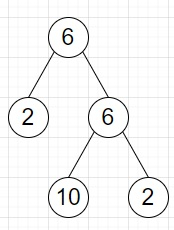# Program to find leaf and non-leaf nodes of a binary tree in Python

Suppose we have a binary tree, we have to find a list of two numbers where the first number is the count of leaves in the tree and the second number is the count of non-leaf nodes.

So, if the input is likethen the output will be (3, 2), as there are 3 leaves and 2 non-leaf nodes.

To solve this, we will follow these steps −

• if n is null, then
• return (0, 0)
• if left of n is null and right of n is null, then
• return (1, 0)
• left := solve(left of n)
• right := solve(right of n)
• return (left + right, 1 + left + right)

Let us see the following implementation to get better understanding −

## Example

Live Demo

class TreeNode:
def __init__(self, data, left = None, right = None):
self.val = data
self.left = left
self.right = right
class Solution:
def solve(self, n):
if not n:
return 0, 0
if not n.left and not n.right:
return 1, 0
left, right = self.solve(n.left), self.solve(n.right)
return left + right, 1 + left + right
ob = Solution()
root = TreeNode(6)
root.left = TreeNode(2)
root.right = TreeNode(6)
root.right.left = TreeNode(10)
root.right.right = TreeNode(2)
print(ob.solve(root))

## Input

root = TreeNode(6)
root.left = TreeNode(2)
root.right = TreeNode(6)
root.right.left = TreeNode(10)
root.right.right = TreeNode(2)

## Output

(3, 2)